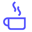a concise shot of dev knowledge
Become a Contributor
Concise shots of dev knowledge

RELATED TAGS

bst
data structures

# What is a Binary Search Tree?Edpresso Team

A Binary Search Tree is a binary tree where each node contains a key and an optional associated value. It allows particularly fast lookup, addition, and removal of items.

The nodes are arranged in a binary search tree according to the following properties:

• The left subtree of a particular node will always contain nodes with keys less than that node’s key.

• The right subtree of a particular node will always contain nodes with keys greater than that node’s key.

• The left and the right subtree of a particular node will also, in turn, be binary search trees.## Time Complexity

In average cases, the above mentioned properties enable the insert, search and deletion operations in $O(log n)$ time where n is the number of nodes in the tree. However, the time complexity for these operations is $O(n)$ in the worst case when the tree becomes unbalanced.

## Space Complexity

The space complexity of a binary search tree is $O(n)$ in both the average and the worst cases.

## Types of Traversals

The Binary Search Tree can be traversed in the following ways:

1. Pre-order Traversal
2. In-order Traversal
3. Post-order Traversal

The pre-order traversal will visit nodes in Parent-LeftChild-RightChild order.

The in-order traversal will visit nodes in LeftChild-Parent-RightChild order. In this way, the tree is traversed in an ascending order of keys.

The post-order traversal will visit nodes in LeftChild-RightChild-Parent order.

RELATED TAGS

bst
data structures
RELATED COURSES

View all Courses

Keep Exploring

Learn in-demand tech skills in half the time